Home > CC1 > Chapter 8 > Lesson 8.3.3 > Problem8-117

8-117.
1. Convert the following data and write equivalent measurements. Use the information in the Math Notes box to help you. Homework Help ✎

1. How many inches are in 6 feet?

2. How many inches are in 5 feet, 2 inches?

3. How many minutes are in 7.5 hours?

4. How many hours are in 2 days?

5. How many days are in 3168 minutes?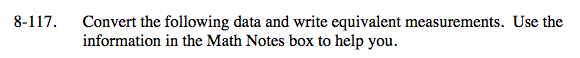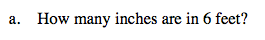1 foot = 12 inches

72 inches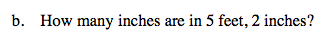1 foot = 12 inches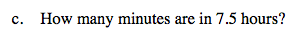1 hour = 60 minutes

450 minutes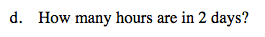1 day = 24 hours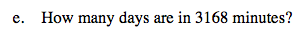1 day = (24 · 60) minutes

2.2 days